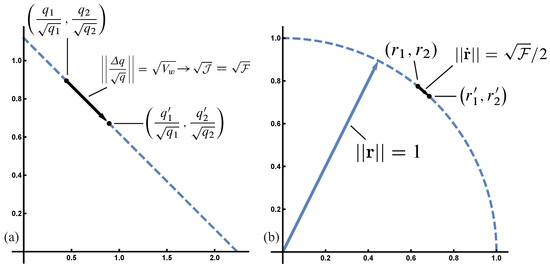Next Article in Journal
Heat Transfer Performance of a Novel Multi-Baffle-Type Heat Sink
Previous Article in Journal
Bayesian 3D X-ray Computed Tomography with a Hierarchical Prior Model for Sparsity in Haar Transform Domain
Open AccessReview

# The Price Equation Program: Simple Invariances Unify Population Dynamics, Thermodynamics, Probability, Information and Inference

bySteven A. FrankDepartment of Ecology and Evolutionary Biology, University of California, Irvine, CA 92697-2525, USA
Entropy 2018, 20(12), 978; https://doi.org/10.3390/e20120978
Received: 22 October 2018 / Revised: 26 November 2018 / Accepted: 14 December 2018 / Published: 16 December 2018
The fundamental equations of various disciplines often seem to share the same basic structure. Natural selection increases information in the same way that Bayesian updating increases information. Thermodynamics and the forms of common probability distributions express maximum increase in entropy, which appears mathematically as loss of information. Physical mechanics follows paths of change that maximize Fisher information. The information expressions typically have analogous interpretations as the Newtonian balance between force and acceleration, representing a partition between the direct causes of change and the opposing changes in the frame of reference. This web of vague analogies hints at a deeper common mathematical structure. I suggest that the Price equation expresses that underlying universal structure. The abstract Price equation describes dynamics as the change between two sets. One component of dynamics expresses the change in the frequency of things, holding constant the values associated with things. The other component of dynamics expresses the change in the values of things, holding constant the frequency of things. The separation of frequency from value generalizes Shannon’s separation of the frequency of symbols from the meaning of symbols in information theory. The Price equation’s generalized separation of frequency and value reveals a few simple invariances that define universal geometric aspects of change. For example, the conservation of total frequency, although a trivial invariance by itself, creates a powerful constraint on the geometry of change. That constraint plus a few others seem to explain the common structural forms of the equations in different disciplines. From that abstract perspective, interpretations such as selection, information, entropy, force, acceleration, and physical work arise from the same underlying geometry expressed by the Price equation. View Full-Text
Show FiguresFigure 1

MDPI and ACS Style

Frank, S.A. The Price Equation Program: Simple Invariances Unify Population Dynamics, Thermodynamics, Probability, Information and Inference. Entropy 2018, 20, 978.